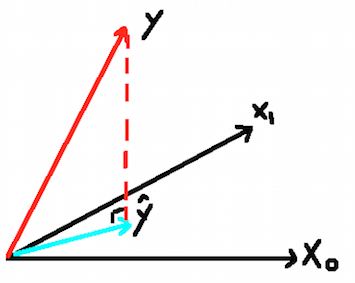# Machine Learning Basics(1): Linear Regression

As a beginner in machine learning, I plan to sketch out my learning process. And it will be my first post in this series.

### 1. Definition

We have an input vector $X^T=(X_1, X_2, \dots, X_p)$ and want to predict a real-valued output $Y$. The linear regression model has the form

Here the $\beta_j$’s are unknown parameters or coefficients.

### 2. Least Squares

Typically we have a set of training data $(x_1,y_1)\dots(x_N,y_N)$ from which to estimate the parameters $\beta$. The most popular estimation method is least squares, in which we pick the coefficients $\beta=( \beta_0, \beta_1, \dots, \beta_p)^T$ to minimize the residual sum of squares (RSS)

How do we minimize $(2.1)$? This is an important question with a beautiful answer. The best $\beta$ can be found by geometry or algebra or calculus. Let’s explain the three approaches one by one.

#### Calculus Interpretation

Denote by $\mathbf{X}$ the $N\times (p+1)$ matrix with each row an input vector (with a 1 in the first position). We can rewrite $(2.1)$ in the matrix form as

This is a quadratic function in the $p+1$ parameters. Differentating with respect to $\beta$ we obtain

In order to get the minimum RSS, we set the first derivative to zero

to obtain the unique solution

The fitted values are

One thing which should be noticed is that it’s wrong to split $(\mathbf{X}^T\mathbf{X})^{-1}$ into $\mathbf{X}^{-1}$ times $\mathbf{X}$ and cancel the terms before $\mathbf{y}$ in $(2.6)$. The matrix $\mathbf{X}$ is rectangualr and has no inverse matrix. And if $\mathbf{X}$ is square and invertible, $\mathbf{\hat y}$ is exactly $\mathbf{y}$ just as $(2.6)$ shows.

Someone may be confused by how to get $(2.3)$ by $(2.2)$ just as I once experienced. Actually, the easiest way to figure it out is to differentiate with respect to each $\beta_j$ respectively

Then, we put all the derivatives together and rewrite it in matrix form to get $(2.3)$.

#### Geometry InterpretationThe picture above shows a direct gemotrical representation of the least squares estimate with two predictors. It’s convenient to extend this to the case with more predictors. We denote the column vectors of $X$ by $\mathbf{x}_0,\mathbf{x}_1,\dots,\mathbf{x}_p$ with $\mathbf{x}_0 \equiv 1$. These vectors span a subspace of $\mathbb{R}^N$, also referred to as the column space of $\mathbf{X}$. We minimize $RSS(\beta)=\lVert\mathbf{y}-\mathbf{X}\beta\rVert^2$ by choosing $\hat \beta$ so that the residual vector $\mathbf{y}-\mathbf{\hat y}$ is orthogonal to this subspace. This orthogonality is expressed in $(2.4)$, and the resulting estimate $\mathbf{\hat y}$ is hence the orthogonal projection of $\mathbf y$ onto this subspace.

#### Algebra Interpretation

In the world of linear algebra, we will also view our problem from a perspective of algebra. Now we reformulate the problem as to solve the linear equations system $\mathbf{X}\beta=\mathbf{y}$. If you are somewhat familar with linear algebra, you can try to use elimination to solve it. Unfortunately, it has no solution typically due to there are more equations than unknowns ($n$ is larger than $p$).

Instead, we hope that the length of error $\mathbf{e}=\mathbf{y}-\mathbf{X}\hat\beta$ is as small as possible. And the corresponding $\hat\beta$ is a least squares solution.

When $\mathbf{X}\beta=\mathbf{y}$ has no solution, we can multiply by $\mathbf{X}^T$ and solve $\mathbf{X}^T\mathbf{X}\beta=\mathbf{X}^T\mathbf{y}$. And it yields the solution from calculus exactly. In the language of algebra, we can split the $\mathbf{y}$ into two parts as $\mathbf{p}+\mathbf{e}$. The part in the column space is $\mathbf{p}$. The perpendicular part in the nullspace of $\mathbf{X}^T$ is $\mathbf{e}$. There is an equation we cannot solve ($\mathbf{X}\beta=\mathbf{y}$). There is an equation $\mathbf{X}\hat\beta=\mathbf{p}$ we do solve. And the solution to $\mathbf{X}\hat\beta=\mathbf{p}$ leaves the least possible error. How do we choose $\mathbf{p}$ in the column space? Just as the geometry part tells us, the projection of $\mathbf{y}$ onto the column space!

Ok, now the three interpretations come together and form a complete picture for the least squares.

Wait a minute, there is still one more question. It might happen that that the columns of $\mathbf{X}$ are not linearly independent, so that $\mathbf{X}$ is not of full rank. Then $\mathbf{X}^T\mathbf{X}$ is singular and the least squares coefficients $\hat\beta$ are not uniquely defined. However, the fitted values $\mathbf{\hat y}=\mathbf{X}\hat\beta$ are still the projection of $\mathbf{y}$ onto the column space of $\mathbf{X}$; there is just more than one way to express that projections in terms of the column vectors of $\mathbf{X}$.

### 3. Implementations

The implementations is extremely easy in a language like R, even without specific packages. The core code is shown below:

You can also use packages like lm in R. It provides not only $\hat\beta$ but also a lot sophisticated coefficients.

To be continued